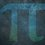# UKMT Special (Problem $15$)

The five-digit number $a679b$ is a multiple of $72$

What are the values of $a$ and $b$?

[UKMT Hamilton Olympiad $2015$, H$1$]Note by Yajat Shamji
8 months, 2 weeks ago

This discussion board is a place to discuss our Daily Challenges and the math and science related to those challenges. Explanations are more than just a solution — they should explain the steps and thinking strategies that you used to obtain the solution. Comments should further the discussion of math and science.

When posting on Brilliant:

• Use the emojis to react to an explanation, whether you're congratulating a job well done , or just really confused .
• Ask specific questions about the challenge or the steps in somebody's explanation. Well-posed questions can add a lot to the discussion, but posting "I don't understand!" doesn't help anyone.
• Try to contribute something new to the discussion, whether it is an extension, generalization or other idea related to the challenge.

MarkdownAppears as
*italics* or _italics_ italics
**bold** or __bold__ bold
- bulleted- list
• bulleted
• list
1. numbered2. list
1. numbered
2. list
Note: you must add a full line of space before and after lists for them to show up correctly
paragraph 1paragraph 2

paragraph 1

paragraph 2

[example link](https://brilliant.org)example link
> This is a quote
This is a quote
    # I indented these lines
# 4 spaces, and now they show
# up as a code block.

print "hello world"
# I indented these lines
# 4 spaces, and now they show
# up as a code block.

print "hello world"
MathAppears as
Remember to wrap math in $$ ... $$ or $ ... $ to ensure proper formatting.
2 \times 3 $2 \times 3$
2^{34} $2^{34}$
a_{i-1} $a_{i-1}$
\frac{2}{3} $\frac{2}{3}$
\sqrt{2} $\sqrt{2}$
\sum_{i=1}^3 $\sum_{i=1}^3$
\sin \theta $\sin \theta$
\boxed{123} $\boxed{123}$

Sort by:

You have until next Monday, $3:00$pm!

- 8 months, 2 weeks ago

Step 1) A number is divisible by 72, if and only if it is divisible by 8 and 9.

Step 2) According to divisibility rules, a679b is divisible by 8 if 79b is divisible by 8.

   Only b = 2 that satisfy.


Step 3) According to divisibility rules, a6792 is divisible by 8 if a + 6 + 7 + 9 + 2 is divisible by 9.

   a + 6 + 7 + 9 + 2 = a + 24 is divisible by 9.
Only a = 3 that satisfy


The five-digit number is 36792.

- 8 months, 2 weeks ago

Correct solution and method!

- 8 months, 2 weeks ago